An Investigation of Various Weighting Schemes for Portfolios | OMICS International
Journal of Business & Financial Affairs

# An Investigation of Various Weighting Schemes for Portfolios

Tamiz M1* and Azmi RA2

1Department of Quantitative Methods and Information Systems, College of Business Administration, Kuwait University, Kuwait

2Middle Eastern Sovereign Wealth Fund, Kuwait

*Corresponding Author:
Professor of Operational Research, Department of Quantitative Methods and Information Systems
College of Business Administration, Kuwait University, P.O. Box 5486, Safat, 13055 Kuwait
Tel: (0965) 97597848
E-mail: [email protected]

Received date: May 20, 2016; Accepted date: July 15, 2016; Published date: July 25, 2016

Citation: Tamiz M, Azmi RA (2016) An Investigation of Various Weighting Schemes for Portfolios. J Bus Fin Aff 5:203. doi: 10.4172/2167-0234.1000203

Copyright: © 2016 Tamiz M, et al. This is an open-access article distributed under the terms of the Creative Commons Attribution License, which permits unrestricted use, distribution, and reproduction in any medium, provided the original author and source are credited.

Visit for more related articles at Journal of Business & Financial Affairs

#### Abstract

This paper investigates methods for finding the proportion of funds (weights) invested in each asset in a portfolio that has been set up based on the decision maker’s choice of assets from a given set. These methods include, equal, ranked and return-based weights as well as Sharpe, Treynor, Markowitz and Goal Programming. The results show that using an optimization methodology produces a more reasonable portfolio with respect to risk and return. This is followed by Sharpe and Treynor methodologies. The weighting schemes experimented in this paper would enable investors to systematically establish the preferred weights for their investment portfolios.

#### Keywords

Weighting; Schemes; Proportions; Goal programming; Optimization; Investors

#### Introduction

Portfolio analysis theory implies that an investor’s problem includes security analysis, portfolio analysis and portfolio selection. Even after completing these tasks, the investor sometimes is required to perform an additional key task, which is to establish portfolio proportions (weights) by using an appropriate weighting scheme. Therefore, weighting schemes could be considered as an integral part of portfolio selection problems.

Some portfolio selection methodologies, such as Sharpe and Treynor, identify the desired assets for the portfolio, but not their weights [1,2]. Some investors may also pick a portfolio purely based on, say, dividend yield, P/E ratio, returns etc.

The weights that are assigned to a given asset in a portfolio can make a contribution to return that is just as important as the asset selection and investment timing decisions . This paper develops and explores several schemes for obtaining portfolio weights. These schemes include, equal and ranking weights, Sharpe, Treynor, returnbased weightings as well as Markowitz and Goal Programming. The results show that using an optimization methodology produces a more reasonable portfolio with respect to portfolios’ risk and return. This is followed by Sharpe and Treynor methodologies which measure the risk adjusted returns.

In general m assets are selected from a set on n and the resulting portfolio is tested over T periods. The experiments reported in this paper are concerned with selecting portfolios of mutual funds and stocks. For the portfolio of mutual funds, ten (n=10) index tracking mutual funds from UK’s financial market based on their net asset value are considered. Their Sharpe Ratios are computed and ranked to select the top five (m=5) funds. For the portfolio of stocks, ten (n=10) stocks from FTSE 100 index are chosen. The stocks are ranked based on their P/E ratios and top five (m=5) are selected. These portfolios are then used for testing and analysis of various weighting schemes developed and explored in the paper.

Some basic algorithms for converting values to weights are stated in section 2. The weighting schemes used in this paper for portfolios of mutual funds and stocks are fully described in section 3. Analysis of the calculated weights of portfolios of mutual funds and stocks are given in sections 4 and 5 respectively. In section 6 some conclusions based on the findings of this paper are given together with some suggestions of further research.

Values and their weights

The following algorithms are used to find the relevant weights of any values used to select portfolio assets. These values maybe positive or negative and represent many financial criteria of relevance to the decision makers’ aims such as returns, dividend yields, P/E ratios, etc. In these algorithms Vi represents the assigned value to the ith asset and Wi represents the resultant weight.

Algorithm (1): All positive values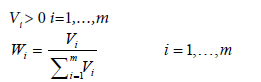Algorithm (2): All negative values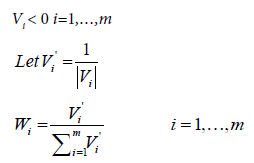This algorithm ensures that the order of importance is preserved (i.e. the smaller the negative value, the larger the weight).

Algorithm (3): For both positive and negative values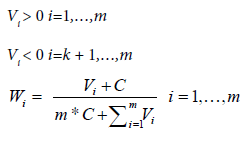Where: C is a large enough positive scaling factor for making all values positive.

Algorithm 4: Ranked assets

Vi > 0, i=1,…,m, and representing the rank of asset i in the set of m assets.

The ith weight is then calculated by: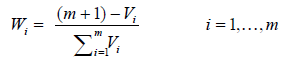#### Weighting Schemes for Portfolios

Equal values

The weights for each asset in portfolios are then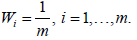Sharpe value

The value of Sharpe Ratio (Sharpe, 1966) for each asset is calculated as follows :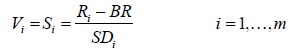Where:

Si=Sharpe ratio of asset i.

Ri=Return of asset i.

BR=Risk free rate (Bank of England interest rate).

SDi=the standard deviation (risk) of asset i.

All Wi are calculated from Vi using algorithms 1, or 2 or 3 in section 2 depending on the signs of Vi

Treynor value

The Treynor Ratios are calculated as follows :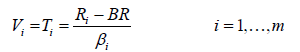Where:

Ti=Treynor Ratio of asset i.

βi=Beta Coefficient, a measure of systematic risk , of the ith constituent.

All Wi are calculated from Vi using algorithms 1, or 2 or 3 in section 2 depending on the signs of Vi

Returns value

This weighting scheme establishes portfolio asset weight based on their average return

Vi=Average rate of return for asset i.

All Wi are calculated from Vi using algorithms 1, or 2 or 3 in section 2 depending on the signs of Vi

Ranking value

Algorithm 4 is used to obtain the portfolio weights, Wi, for all the ranked assets in the portfolio.

GP weight

Goal Programming (GP) is perhaps the most widely used approach in the field of multiple criteria decision making that enables the decision maker to incorporate numerous variations of constraints and goals [5-9]. Original Portfolio Selection problem, with two factors of risk and return can be viewed as a Goal Programming with two objectives . GP models can also be constructed for the analysis of Mutual Funds with several factors .

In this paper, two GP models are used for establishing the weights of each asset in the portfolios; one for comparison with other portfolios and the second for comparison with the benchmark. In both models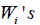(the weights) are restricted to take a value of at least 1/m so that all m assets are selected purposes.

Mutual funds: The GPMF1 model for the mutual funds is as follows: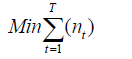Subject to: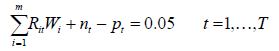(1)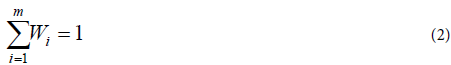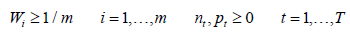Where:

nt is the negative deviational variable.

pt is the positive deviational variable.

Constraint 2 above ensures that the entire fund is invested in the portfolio.

This model is set up to maximize the portfolio’s return. The target values in (1) are all set at 5% which is considered realistic returns for mutual funds. If, however, the target values in (1) are set to other values for each period to better reflect the return of the resultant portfolio, then a normalization method may need to be used to ensure all target values are measured in the same unit of measurement .

The GPMF2 model for the mutual funds based on the Konno’s model is as follows :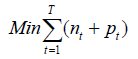Subject to: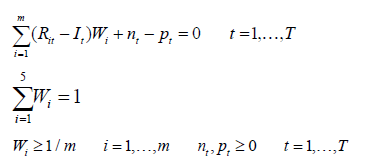Where:

It=Return of the index (FTSE 100) during period t.

Stocks: Two GP models are proposed for selecting the proportions of each stock in the portfolio. GPS1 model is for comparison with other portfolios, while GPS2 is for comparison with the benchmark.

The GPS1 model for the stocks portfolio is as follows: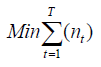Subject to: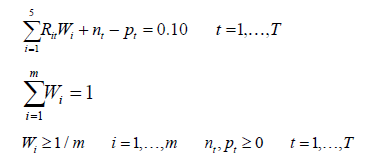The GPS2 model for the stocks portfolio is as follows (GPS2 model is maximizing return relative to index for the m stocks):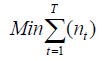Subject to: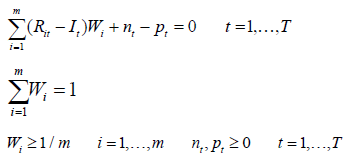Markowitz weight

The Markowitz optimization model can be utilized for deciding on asset weights as follows :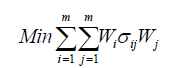Subject to: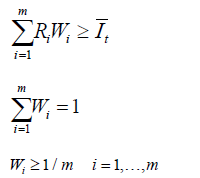Where: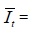The average return of the index (FTSE 100) during period t.

The proportions are restricted to take a value of at least 1/m so that all m assets are selected for Mutual Funds and Stocks portfolios.

Market cap value

The weight of each stock is obtained using the value of market capitalization of each stock.

Vi=Market Cap for asset i.

As all Vi are positive, algorithm 1 is used to calculate the corresponding weight for each stock.

#### Comparison between the Weighting Schemes for Mutual Fund Portfolios

As stated above, based on net asset value, ten index tracking mutual funds from UK’s financial market are considered. Their Sharpe Ratios are computed and ranked from the highest to the lowest [1,15]. The following table contains the ten mutual funds, their Sharpe ratio and their ranks (Table 1):

Mutual Fund Name HE AR SU JP AN FC II GA FI AI
Sharpe Ratio -1.33 -1.38 -1.41 -1.43 -1.46 -1.48 -1.48 -1.67 -1.67 -1.68
Sharpe Ranking(1 is the best,10 is the worst) 1 2 3 4 5 6 7 8 9 10

Table 1: The sharpe ratio and ranking of 10 mutual funds.

The top 5 assets are used for the mutual funds portfolio. Their corresponding weights are then calculated using different weighting schemes during the time period of consideration which has a total of 105 observations over 21 weeks (t=1,…, 21).

Table 2 contains all the values obtained from Sharpe and Treynor methods as well as the average returns for the selected assets in the mutual funds portfolio (Table 2).

Portfolio constituents Vi Constituents’ Values Mutual Fund Portfolios Weighting Schemes
Sharpe Ratio Treynor Ratio Average Return
HE V1= -1.33 -0.076 -0.90
AR V2= -1.38 -0.067 -0.48
SU V3 = -1.41 -0.074 -0.77
JP V4 = -1.43 -0.069 -0.44
AN V5 = -1.46 -0.072 -0.53

Table 2: Various ratios for mutual fund portfolios.

Note, all the values for Sharpe, Treynore and average return in Table 2 are negative, thus their corresponding portfolio weights are calculated using algorithm 2.

Table 3 contains all the weights obtained from various weighting schemes discussed in section 3 (Table 3).

Portfolio constituents Vi
Constituents’ Proportions
Mutual Fund Weighting Schemes (%)
Equal Weight Sharpe Weight TreynorWeight Return Weight Ranking Weight GPMF1 Weight GPMF2 Weight Markowitz Weight
HE W1= 20.00 21.06 18.89 12.81 33.33 40.00 15.00 40.00
AR W2 = 20.00 20.33 21.40 24.17 26.67 15.00 15.00 15.00
SU W3 = 20.00 19.89 19.43 15.04 20.00 15.00 15.00 15.00
JP W4 = 20.00 19.57 20.53 26.17 13.33 15.00 40.00 15.00
AN W5 = 20.00 19.16 19.84 21.80 6.67 15.00 15.00 15.00

Table 3: Mutual fund portfolios weighting.

The resultant portfolios are compared to each other using the average return, total risk and Sharpe ratio as shown in Table 4:

Portfolio Comparison Weighting Schemes
Equal Weight Sharpe Weight Treynor
Weight
Return Weight Ranking Weight GPMF1 Weight Markowitz Weight
Average portfolio Return 0.56 0.57 0.55 0.48 0.72 0.77 0.77
Total Risk 0.0329 0.0327 0.0333 0.0383 0.0306 0.0292 0.0292
Sharpe Ratio 15.6 16.0 15.1 11.3 22.0 24.7 24.7

Table 4: The average return, total risk and Sharpe ratio of portfolios based on various weighting schemes.

Return and risk are used to compare portfolios against each other. The risk is measured by the standard deviation which measures how much return on a portfolio is deviating from the average returns. Table 4 illustrates that both the GPMF1-weighted portfolio and the Markowitz-weighted portfolio are the ones with the highest rate of return, compared to other portfolios, and moreover they are the two portfolios that have the lowest risk level amongst all other portfolios.

The table shows too that the return-based portfolio has the lowest return which is associated with the highest risk.

When comparing average returns with total risks for all portfolios, the GPMF1-weighted portfolio and the Markowitz-weighted portfolio provide the best compromise between risk and return. The Sharpe ratio is used to establish whether the investor’s return is due to smart investing decisions or the result of taking excess risk. The following table compares the portfolios with their benchmark using the tracking error (Table 5).

Portfolio Comparison Weighting Schemes
Equal Weight Sharpe Weight Treynor
Weight
Return Weight Ranking Weight GPMF2 Weight Markowitz Weight
Average portfolio Return 0.56 0.57 0.55 0.48 0.72 0.49 0.77
Average Benchmark return 0.16
The Tracking Error 0.2147 0.2159 0.2137 0.2076 0.2314 0.2092 0.2395

Table 5: The tracking error of the portfolios based on various weighting schemes.

If the investment goal is to keep track of a relevant benchmark (so as to avoid excessive risk), the best portfolio to select is the one that has the lowest tracking error. Table 5 shows that the return-weighted portfolio is the best portfolio in tracking the benchmark with a tracking error of 0.2076 and a return of 0.48, followed by the GPMF2-weighted portfolio with a tracking error of 0.2092 and a return of 0.49 (higher than the return-weighted portfolio’s return).

#### Comparison between the Weighting Schemes for Stock Portfolios

The following table shows the ten stocks, their P/E ratio and their ranking (Table 6):

The Stock name HS TR PE BH UN RT RD VO GS LB
P/E 158.1 19.7 18.3 18.2 17.9 17.3 13.4 12.8 11.4 1.7
P/E Ranking
(1 is the best,
10 is the worst)
1 2 3 4 5 6 7 8 9 10

Table 6: The 10 stocks ranked based on their P/E ratio.

The top 5 assets are used in the stocks portfolio. Their corresponding weights are calculating using different weighting schemes during the time period under consideration which has a total of 105 observations over 21 weeks (t=1,…, 21).

Table 7 contains all the values obtained from Sharpe and Treynor methods as well as the average returns and the market capitalization for the selected assets in the stocks portfolio (Table 7).

Portfolio constituents Vi
Constituents’ Values
Stock Portfolios Weighting Schemes
Sharpe Ratio Treynor Ratio Average return Market Cap Weight
(Market Cap: Billions of GBP)
HS V1 = -1.07 -0.061 -0.005 123.84
TR V2 = 0.05 0.050 0.096 19.40
PE V3 = -1.14 -0.078 -0.003 8.02
BH V4 = -0.48 -0.025 0.003 134.42
UN V5 = -1.08 -0.082 0.001 61.08

Table 7: Values in various Stock related measurements.

Note, all the values in Table 7 for Sharpe, Treynor and average return are both positive and negative, thus their corresponding portfolio weights are calculated using algorithm 3. The values for market capitalization are all positive, thus algorithm 1 is used for calculating its portfolio weights. Table 8 contains all the weights obtained from various weighting schemes discussed in section 3 (Table 8).

Portfolio constituents Wi
Constituents’ Proportions
Stock Weighting Schemes (%)
Equal Weight Sharpe Weight Treynor
Weight
Return Weight Ranking Weight GPMF1 Weight GPMF2 Weight Markowitz Weight Market Cap Weight
HS W1 = 20.00 3.69 19.45 15.91 33.33 15.00 16.27 15.00 35.71
TR W2 = 20.00 59.90 22.29 33.51 26.67 40.00 38.99 15.00 5.60
PE W3 = 20.00 0.16 19.00 16.33 20.00 15.00 21.21 29.50 2.31
BH W4 = 20.00 33.32 20.35 17.35 13.33 15.00 4.70 15.00 38.76
UN W5 = 20.00 2.93 18.91 16.90 6.67 15.00 18.82 25.50 17.62

Table 8: Stock weighs in various portfolios.

The following table compares the various weighting schemes based on their portfolios’ average return, total risk and Sharpe ratio (Table 9).

Portfolio Comparison Weighting Schemes
Equal Weight Sharpe Weight Treynor
Weight
Return Weight Ranking Weight GPS1 Weight Markowitz Weight Market Cap Weight
Average portfolio Return 0.45 0.97 0.48 0.56 0.59 0.61 0.26 0.65
Total Risk 0.039 0.044 0.039 0.038 0.047 0.038 0.034 0.053
Sharpe Ratio 10.3 20.9 11.1 13.5 11.5 14.8 6.2 11.4

Table 9: The average return, total risk and Sharpe ratio of portfolios based on various weighting schemes.

Table 9 shows return, risk and Sharpe ratio for all portfolios, in which the Sharpe-weighted portfolio is the one with the highest rate of return, compared to other portfolios, followed by market cap and Goal Programming (GPS1 model) weighted portfolios. However, Markowitz-weighted portfolio is the one with the lowest total risk, compared to other portfolios, whereas, the Market Cap-weighted portfolio has the highest risk. When comparing average returns with total risks for all portfolios, the Sharpe-weighted portfolio provides the best compromise between risk and return. The Sharpe ratio is used to establish whether the investor’s return is due to sound investing decisions or the result of taking excess risk. The following table compares the portfolios with their benchmark using the tracking error (Table 10).

Portfolio Comparison Weighting Schemes
Equal Weight Sharpe Weight Treynor
Weight
Return Weight Ranking Weight GPS2 Weight Markowitz Weight Market Cap Weight
Average portfolio Return 0.45 0.97 0.48 0.56 0.59 0.49 0.26 0.65
Average Benchmark return 0.16
The Tracking Error 0.197 0.390 0.197 0.200 0.262 0.183 0.212 0.331

Table 10: The tracking error of the portfolios based on various weighting schemes.

If the investment goal is to keep track of a relevant benchmark, the best portfolio would be the one that has the lowest tracking error with the relevant benchmark. Table 10 shows that the GPS2-weighted portfolio is the best portfolio in tracking the benchmark with a tracking error of 0.183, followed by the Treynor-weighted portfolio and surprisingly the equal-weighted portfolio (albeit GPS2-weighted and Treynor-weighted portfolios have higher return compared to the equally-weighted portfolio).

#### Conclusion and Further Research

This paper explores several weighting schemes for establishing portfolio weights. Applications to mutual funds and stocks are investigated in distinguishable experiments in order to have meaningful comparisons and to validate the results using different asset classes.

The weighting schemes comparisons show that using an optimization methodology produces a more reasonable portfolio weights when considering risk and return. This is particularly true for weighting schemes in mutual fund portfolios, where Goal Programming and Markowitz weighting schemes achieve the best return and risk compared to other portfolios. However, in the experiments of weighting schemes in stock portfolios, Sharpe and Goal Programming weighting schemes achieve the best results compared to other portfolios. The equal-weighting scheme produces the same level of tracking error as that achieved by the Treynor-weighted portfolio, but with less return.

Overall, the best weighting schemes, in mutual funds experiments, in terms of risk adjusted returns, are the Goal Programming and Markowitz weighting schemes (with a Sharpe ratio of 24.7 for each of them), followed by Ranking and Sharpe weights (with Sharpe ratios of 22 and 16, respectively). However, when comparing the resulting portfolios with the relevant benchmark, return and Goal Programming weights are the best with the lowest tracking errors of 0.208 and 0.209 respectively, followed by Treynor and Equal weights.

The results of weighting schemes with applications to stocks imply that Sharpe and Goal Programming weighting schemes are the best ones compared to others in terms of risk adjusted returns, followed by return and ranking weights. Goal Programming is further the weighting scheme with the lowest tracking error of 0.183, followed by Treynor and Equal weights (each with a tracking error of 0.197), and then followed by Return and Markowitz weights.

In this paper the Sharpe ratio is used to select the initial five mutual funds and the P/E ratios are used to select the initial 5 stocks. The weighting schemes are subsequently applied to calculate the respective proportions in each portfolio. Clearly future research warrants further investigation of these or similar weighting schemes based on other selection criteria for the initial assets.

Finally, this paper provides many choices of weighting schemes for investors to systematically explore in order to establish the preferred weights for investment portfolios. Some of the weighting schemes experimented in this paper should assist in stimulating further research and innovation in obtaining systematic ways for deciding on portfolio constituents’ weights.

#### References

Select your language of interest to view the total content in your interested language

### Article Usage

• Total views: 10350
• [From(publication date):
September-2016 - Feb 27, 2020]
• Breakdown by view type
• HTML page views : 10187Can't read the image? click here to refresh